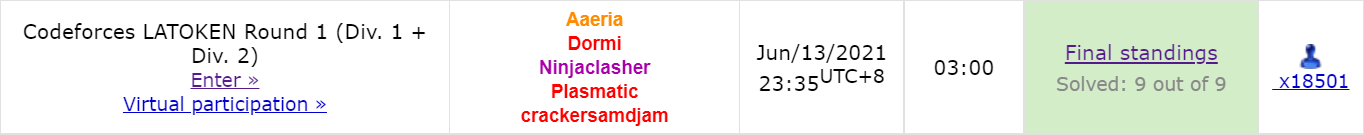E

做法一（我的做法）

(1) $kq\equiv n(\mod 2)$
(2) 询问次数最大的一个数的询问次数不超过 $q$. 即，$n$ 个和为 $kq$ 的奇数，最大的一个数的最小可能值不超过 $q$. 这一点很容易用除法判断。

H

$f[u]$ 表示要确定 $u$ 子树内的一个点，最坏情况下最少要询问多少次。一个很自然的想法是转移枚举第一个询问的是子树里的哪个点，然后分成好多棵子树去处理——但是这种思路其实不太合适，因为枚举一个点再枚举它到根路径上每个点的 DP 值会带来很高的复杂度。不妨这样考虑：我们直接枚举第一个询问的是哪个子树里的点！设这个子树是 $c_0$，如果答案在其中，那么付出的代价是 $f[c_0]$. 否则，我们花费了 $1$ 次询问排除了这个子树，于是代价等于剩下部分的 $f$$+1$. 对于剩下部分的 $f$ 值，我们继续枚举第一个询问的是哪个子树里的点……由此思考下去，可以发现其实我们在确定一个询问的顺序 $c_0,c_1,...,c_{k-1}$，而最终的 $f$ 值就等于 $\max(f[c_0],f[c_1]+1,f[c_2]+2,...,f[c_{k-1}]+k-1,k)$，我们希望最小化这个值。所以这个顺序就应该是按 $dp$ 值从大到小排序，这样就完成了转移。